Select Page

# MCQ Probability CBSE Maths 12 Science Answers in Hindi

MCQ Probability CBSE Maths 12 Science Answers in Hindi to enable students to get Answers in a narrative video format for the specific question.

Expert Teacher provides MCQ Probability CBSE Maths 12 Science Answers through Video Answers in Hindi language. This video solution will be useful for students to understand how to write an answer in exam in order to score more marks. This teacher uses a narrative style for a question from Probability not only to explain the proper method of answering question, but deriving right answer too.

Please find the question below and view the Answer in a narrative video format.

Question:

## Similar Questions from CBSE, 12th Science, Maths, Probability

Question 1 : If E and F are two events such that,, Find P(not E and not F). (View Answer Video)

Question 2 : If P(A)=0.8, P(B)=0.5 and=0.4, find. (View Answer Video)

Question 3 : If P(A)=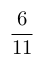, P(B)=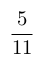and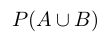=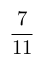, find. (View Answer Video)

Question 4 : Compute P(A/B) if P(B) =0.5 and P(A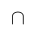B)=0.32. (View Answer Video)

### Application of Derivatives

Question 1 : The normal to the curvepassing (1, 2) is : (View Answer Video)

Question 2 : Which of the following functions are strictly decreasing on(View Answer Video)

Question 3 : The point on the curvewhich is nearest to the point (0, 5) is  (View Answer Video)

Question 4 : The slope of the tangent to the curveat the point (2, -1) is : (View Answer Video)

Question 5 : The slope of the normal to the curveat x = 0 is :
Question 2 : Write the degree of the differential equation :(View Answer Video)
Question 3 : Write the degree of the differential equation(View Answer Video)
Question 4 : Write the degree of the differential equation :(View Answer Video)
Question 5 : Write the degree of the differential equation(View Answer Video)